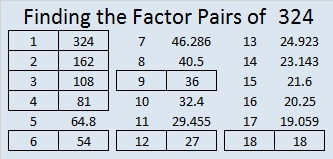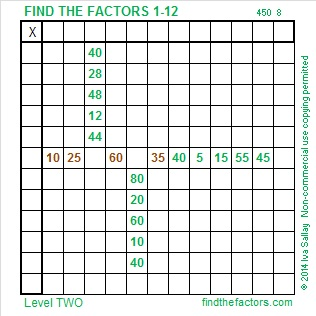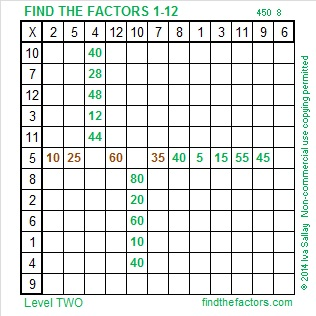# 324 Christmas Factor Trees

• 324 is a composite number.
• Prime factorization: 324 = 2 x 2 x 3 x 3 x 3 x 3, which can be written 324 = (2^2) x (3^4)
• The exponents in the prime factorization are 2 and 4. Adding one to each and multiplying we get (2 + 1)(4 + 1) = 3 x 5 = 15. Therefore 324 has exactly 15 factors.
• Factors of 324: 1, 2, 3, 4, 6, 9, 12, 18, 27, 36, 54, 81, 108, 162, 324
• Factor pairs: 324 = 1 x 324, 2 x 162, 3 x 108, 4 x 81, 6 x 54, 9 x 36, 12 x 27, or 18 x 18
• 324 is a perfect square. √324 = 18Included in these 324 factor trees are smaller factor trees for the numbers in brown: 4, 6, 9, 12, 18, 27, 36, 54, 81, 108, and 162. Prime factors of 324 are in red.This Level 2 puzzle looks like part of a Christmas tree branch. It’s not too difficult to solve. Give it a try!Print the puzzles or type the factors on this excel file: 12 Factors 2014-12-15